Nilpotent element

An elementof a ring or semi-group with zerosuch that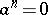for some natural number. The smallest suchis called the nilpotency index of. For example, in the residue ring modulo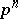(under multiplication), whereis a prime number, the residue class ofis nilpotent of index; in the ring of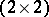-matrices with coefficients in a fieldthe matrixis nilpotent of index 2; in the group algebra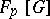, where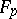is the field withelements andthe cyclic group of ordergenerated by, the element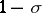is nilpotent of index.

Ifis a nilpotent element of index, then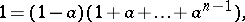that is,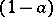is invertible inand its inverse can be written as a polynomial in.

In a commutative ringan elementis nilpotent if and only if it is contained in all prime ideals of the ring. All nilpotent elements form an ideal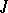, the so-called nil radical of the ring; it coincides with the intersection of all prime ideals of. The ring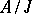has no non-zero nilpotent elements.

In the interpretation of a commutative ringas the ring of functions on the space(the spectrum of, cf. Spectrum of a ring), the nilpotent elements correspond to functions that vanish identically. Nevertheless, the consideration of nilpotent elements frequently turns out to be useful in algebraic geometry because it makes it possible to obtain purely algebraic analogues of a number of concepts in analysis and differential geometry (infinitesimal deformations, etc.).